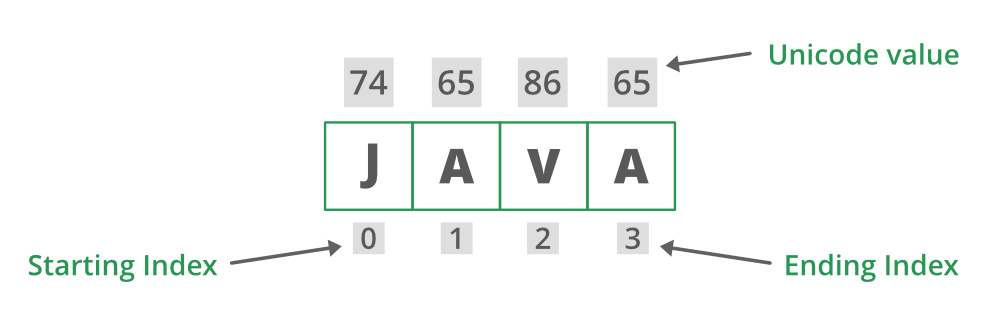# Java Program to Determine the Unicode Code Point at Given Index in String

• Last Updated : 21 Apr, 2022

ASCII is a code that converts the English alphabets to numerics as numeric can convert to the assembly language which our computer understands. For that, we have assigned a number against each character ranging from 0 to 127. Alphabets are case-sensitive lowercase and uppercase are treated differently. Complete ASCII values table can be interpreted but better it is sticking to the table in the illustration below where no need arises to lean complete ASCII values in a table. Extremities are assigned as follows so as order to guess the Unicode value correctly with memorizing the complete table. With this table, one can extract all alphabets Unicode be it uppercase or lowercase.illustration:

From this, one can get Unicode of other values such as B is 66 because lowercase starting is a for which is illustrated from the above table, its 65. For ‘b’ it’s 98. Similarly, for Unicode of ‘G’ is 71, ‘E’ is 69, ‘K’ is 75, and so on.

codePointAt() inbuilt method is used where the user wants to return the character at the specific index. The index refers to character values (Unicode units)  and ranges from 0 to length()-1

Definition: This is an inbuilt function that Returns the character(Unicode point) at the specific index. The index refers to character values (Unicode units) and ranges from 0 to length()-1.

Syntax:

`java.lang.String.codePointAt();`

Parameter: The index to the character values.

Return Type: This method returns the Unicode value at the specified index. The index refers to char values (Unicode code units) and ranges from 0 to [length()-1]. Simply in layman language, the code point value of the character at the index.

Implementation: There are two examples being discussed for clear understanding considering both the cases where there is the involvement of exception with this function and another one simply depicting the internal use of the function.

Example 1: In this edge case of exception handling is not taken into consideration which above method do throws.

## Java

 `// Importing Files and Classes` `import` `java.io.*;`   `class` `GFG {`   `    ``// Main driver method` `    ``public` `static` `void` `main(String[] args)` `    ``{` `        ``// Considering random string for input` `        ``String str = ``"GEEKS"``;`   `        ``// Unicode at index 0` `        ``// Input is a small string` `        ``int` `result_1 = str.codePointAt(``0``);` `        ``int` `result_2 = str.codePointAt(``1``);` `        ``int` `result_3 = str.codePointAt(``2``);` `        ``int` `result_4 = str.codePointAt(``3``);` `        ``int` `result_5 = str.codePointAt(``4``);`   `        ``// Printing the input string` `        ``System.out.println(``"Original String : "` `+ str);`   `        ``// Prints unicode character at index 0 to 4` `        ``// in above input string` `        ``// to show usage of codePointAt()` `        ``System.out.println(``"unicode point at 0 = "` `                           ``+ result_1);` `        ``System.out.println(``"unicode point at 1 = "` `                           ``+ result_2);` `        ``System.out.println(``"unicode point at 2 = "` `                           ``+ result_3);` `        ``System.out.println(``"unicode point at 3 = "` `                           ``+ result_4);` `        ``System.out.println(``"unicode point at 4 = "` `                           ``+ result_5);` `    ``}` `}`

Output:

```Original String : GEEKS
unicode point at 0 = 71
unicode point at 1 = 69
unicode point at 2 = 69
unicode point at 3 = 75
unicode point at 4 = 83```

Time Complexity O(n) of the above code.

Now considering the exception concept here in play, the exception is simply a problem that arises during runtime disrupting the normal flow of the program. They can be of two types checked exceptions and unchecked exceptions. Checked can be detected by our compiler where unchecked exceptions can not be detected by the compiler. For this sake, exception handling techniques in java to deal with the same. Now dealing with the exception in the function. Sometimes an exception is thrown when an index that is beyond memory is being tried to access. Below are the conceptual details about the exceptions in codePointAt() method. Likewise, discussing IndexOutOfbound exception downside and in order to deal with it try-catch technique.

Example 2: IndexOutOfBoundsException is thrown to indicate that an index of some sort (such as to an array, to a string, or to a vector) is out of range is as follows. Below is the example to illustrate codeAtPoint() where the exception is thrown.

## Java

 `import` `java.io.*;`   `class` `GFG {`   `    ``// Main driver method` `    ``public` `static` `void` `main(String[] args)` `    ``{`   `        ``// Try block to check exceptions` `        ``try` `{`   `            ``// Input string` `            ``String str = ``"Geeksforgeeks"``;`   `            ``// unicode at index 0` `            ``// Storing it in integer variable` `            ``int` `result_1 = str.codePointAt(``0``);`   `            ``// unicode at index 4` `            ``int` `result_2 = str.codePointAt(-``4``);`   `            ``// Printing input/original string` `            ``System.out.println(``"Original String : "` `+ str);`   `            ``// Prints unicode character at index 1 in string` `            ``System.out.println(``"Character(unicode point) = "` `                               ``+ result_1);`   `            ``// Prints unicode character at index 4 in string` `            ``System.out.println(``"Character(unicode point) = "` `                               ``+ result_2);` `        ``}`   `        ``// Catch block to handle exception` `        ``catch` `(IndexOutOfBoundsException e) {`   `            ``// Message printed if exception occurs` `            ``System.out.println(``"Exception thrown  :"` `+ e);` `        ``}` `    ``}` `}`

Output

`Exception thrown  :java.lang.StringIndexOutOfBoundsException: index -4,length 13`

My Personal Notes arrow_drop_up
Recommended Articles
Page :